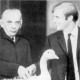# Gracious Living

Products of Groups
November 19, 2010, 22:49
Filed under: Algebra, Math | Tags: , , , ,

Ugh, so, I’ve been really busy today and haven’t had the time to do a Banach-Tarski post.  Since I really do want to see MaBloWriMo to the end, I’m going to take a break from the main exposition and quickly introduce something useful.  There are a couple major ways of combining two groups into one.  The most important one, called the direct product, is analogous to the product of topological spaces.  I know this is sort of a wussy post — sorry.

The easiest construction is the direct product.  Let$G_i$ be a family of groups indexed by$i\in I$.  Then the direct product of$G_i$,$\prod_{i\in I}G_i$, is the group whose underlying set is the Cartesian product of the underlying sets of$G_i$, with multiplication defined componentwise.  Thus, every element of$\prod G_i$ is an$I$-tuple$(g_i)_{i\in I}$, with$(g_i)(h_i)=(g_ih_i)$.

Like the product topology, this has a universal property that characterizes it: it has a canonical projection homomorphism$p_i$ down to each$G_i$, and any other group$H$ that projects to each$G_i$ with maps$q_i$ factors through the direct product: we can write$q_i=p_i\circ r$ for some$r:H\rightarrow\prod G_i$.  This is pretty easy to prove: let$r$ be the map that sends$h\in H$ to the tuple whose$i$th coordinate is$q_i(h)$.  Then$p_ir(h)=q_i(h)$ by definition.

Since groups have more structure than topological spaces, there are also injections$G_i\rightarrow\prod G_i$ sending$g\in G_i$ to the tuple whose$i$th coordinate is$G$ and all of whose other coordinates are identities.  But the direct product doesn’t have the universal property for these injections.  We’ll see a construction that does below.  One way of noticing this is by seeing that the images of these injections don’t generate$\prod G_i$ (since we defined generation in terms of finite products): they only generate the subgroup of$\prod G_i$ whose elements have identities in all but finitely many of their coordinates.

Now, fortunately for our purposes, infinite products of groups are a lot less important than infinite products of topological spaces.  More often, we’ll be working with finite products, and can rely on simple observations like$G\times H=\{(g,h):g\in G,h\in H\}$ with$(g_1,h_1)(g_2,h_2)=(g_1g_2,h_1h_2)$.  As expected,$(G\times H)\times J$ is uniquely isomorphic to$G\times (H\times J)$, etc., etc.

One thing of note is that$G\times H$ contains both$G$ and$H$ as normal subgroups.  (Check this.)  In fact, there’s a way to go backwards: let$G,H$ be normal subgroups of$J$ with$G\cap H=\{e\}$,$GH=J$.  Then$J\cong G\times H$.  Let’s prove this.  The second condition allows us to write any element of$J$ as a product$gh,g\in G,h\in H$.  Now suppose that$j=g_1h_1=g_2h_2$; then$g_2^{-1}g_1=h_2h_1^{-1}$.  Since$G\cap H=\{e\}$, both products must be$e$, so$g_1=g_2,h_1=h_2$: any element of$J$ is a unique product of an element of$G$ and an element of$H$.  This shows that$J\cong G\times H$ as sets.

But this isn’t enough, yet.  Let’s look at the element$ghg^{-1}h^{-1}$, called the commutator of$g$ and$h$, since it is equal to the identity if and only if$g$ and$h$ commute.  We have$ghg^{-1}h^{-1}\in G(hGh^{-1})=GG=G$, and similarly$ghg^{-1}h^{-1}\in (gHg^{-1})H=HH=H$, so it is in$G\cap H$, so it is the identity.  Thus, every element of$G$ commutes with every element of$H$.  In particular,$g_1h_1g_2h_2=g_1g_2h_1h_2$, which is precisely the multiplication of the direct product.  Thus,$J\cong G\times H$.

As an exercise, prove that$\mathbb{Z}_m\times\mathbb{Z}_n\cong\mathbb{Z}_{mn}$ when$m$ and$n$ are relatively prime (they have no common factors other than$1$.

1 Comment so far